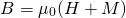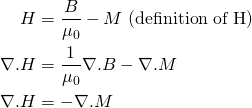# H field and material boundaries

For a long time I thought of the H field as being generated by free currents only. The problem with this view is that it leads us to make erroneous assumptions. We know from the definition of H that:The magnetization M is 0 outside of a material. So if we assume that H is due to free currents only, one would conclude that the magnetic field/flux B outside a material is also a function of free currents only – e.g. the external magnetic field of a solenoid would be independent of the material the core is made of. However, as seen in my previous post, this is not true. Also, one might conclude that permanent magnets have no external magnetic field, which is clearly not true either…!

Actually while ∇.B = 0 everywhere (there are no magnetic monopoles), ∇.H ≠ 0. The H field has sources wherever ∇.M ≠ 0:

(1)In other words if we want to calculate the magnitude of the H field throughout space, not only do we need to consider free currents (which produce circulation in the H field), but also material boundaries and other areas where M is non-uniform (producing sources in the H field).

Nonetheless, in typical elementary problems where we integrate around a closed loop, only the curl is important and we can still write for example ∮H.dl = Ipiercing (plus the dE/dt term if relevant).

Props to Griffiths’ Introduction to Electrodynamics section 6.3.2 for helping to clearing up this confusion in my mind.

This entry was posted in Physics. Bookmark the permalink.